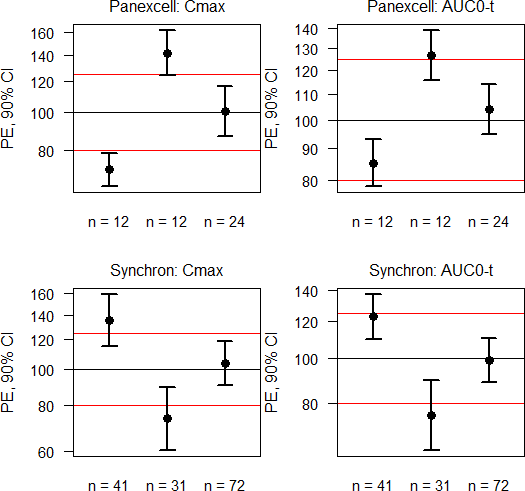## Visualization [GxP / QC / QA]

Hi ElMaestro,

» […] it makes very good sense to plot for example the RMSE, CV, SE of diff., or even the width of the CI as function of (cumulated) number of subjects.

If you have all data, of course. A simple visualization using the res data.frame of my previous post:windows(width = 5.5, height = 5.5) op <- par(no.readonly = TRUE) par(mar = c(3, 4, 2, 0), cex.axis = 0.9, cex.main = 1, font.main = 1) split.screen(c(length(CRO), length(metric))) scrn <- 0 for (j in seq_along(CRO)) {   for (k in seq_along(metric)) {     scrn <- scrn + 1     screen(scrn)     plot(c(0.5, length(study)+0.5), rep(100, 2), type = "n",          ylim = range(res$lower[res$CRO == CRO[j] & res$metric == metric[k]], res$upper[res$CRO == CRO[j] & res$metric == metric[k]]),          log = "y", xlab = "", ylab = "PE, 90% CI", axes = FALSE,          main = paste0(CRO[j], ": ", metric[k]))     axis(2, las = 1)     axis(1, at = seq_along(study), tick = FALSE,          labels = paste("n =", res$n[res$CRO == CRO[j] &                                res$metric == metric[k]])) abline(h = c(80, 100, 125), col = c("red", "black", "red")) box() for (l in seq_along(study)) { arrows(x0 = l, y0 = res$lower[res$CRO == CRO[j] & res$metric == metric[k] &                             res$study == study[l]], y1 = res$upper[res$CRO == CRO[j] & res$metric == metric[k] &                             res$study == study[l]], length = 0.08, angle = 90, lwd = 2, code = 3) points(l, res$PE[res$CRO == CRO[j] & res$metric == metric[k] &                        res\$study == study[l]], pch = 19, cex = 1.25)     }   } } close.screen(all = TRUE) par(op)

Dif-tor heh smusma 🖖Helmut SchützThe quality of responses received is directly proportional to the quality of the question asked. 🚮
Science Quotes22,305 posts in 4,668 threads, 1,587 registered users;
online 5 (0 registered, 5 guests [including 2 identified bots]).
Forum time: Thursday 22:46 CEST (Europe/Vienna)

On two occasions I have been asked,—“Pray, Mr. Babbage,
if you put into the machine wrong figures,…
will the right answers come out?”
…
I am not able rightly to apprehend the kind of confusion of ideas
that could provoke such a question.    Charles Babbage

The Bioequivalence and Bioavailability Forum is hosted byIng. Helmut Schütz